Pure Bending

Kinematics of pure bending:

When a bar is subjected to a pure bending moment as shown in the figure it is observed that axial lines bend to form circumferential lines and transverse lines remain straight and become radial lines.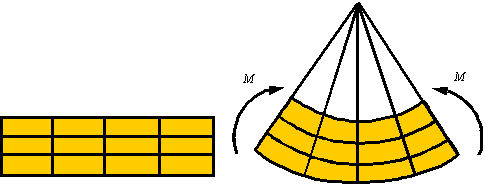In the process of bending there are axial line that do not extend or contract. The surface descrived by the set of lines that do not extend or contract is called the neutral surface. Lines on one side of the neutral surface extend and on the other contract since the arc length is smaller on one side and larger on the other side of the neutral surface. The figure shows the netral surface in both the initial and the bent configuration.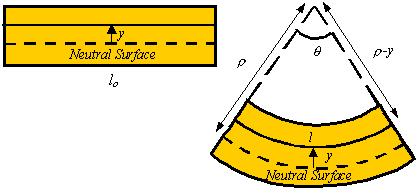The axial strain in a line element a distance y above the neutral surface is given by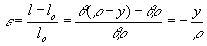where r is the radius to the neutral surface.

Stress distribution in pure bending:

By Hooke’s law, the axial stress is given in terms of the axial strain by the relation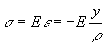Therefore, the axial stress is zero on the neutral surface and increases linearly as one moves away from the neutral axis.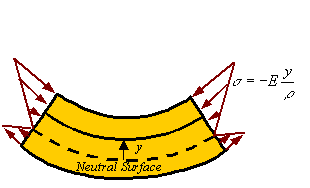Axial load and the location of the neutral axis: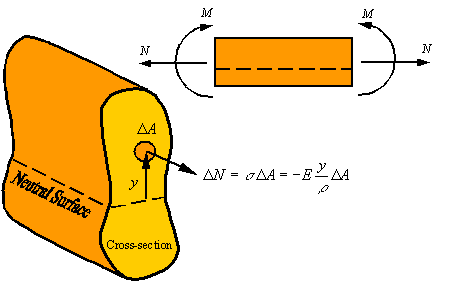There is zero axial load in a member under pure bending. Therefore, the axial load generated by the stress should be zero. The axial load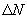generated by the stress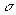applied on the area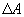of the cross section is given by the approximate relation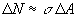The total load on the cross section can be calculated by integrating this relation over the cross section. This yields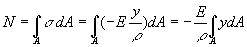Since the axial load is zero during pure bending, one concludes that for pure bending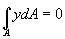The reader recalls that the location of the centroid of an area is calculated from the relation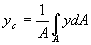Therefore, for the axial load to be zero, the neutral axis must pass through the centroid of the cross section (i.e., yc=0). In the event that the axial load is not zero, the location of the neutral axis relative to the centroid of the cross section can be calculated from the relation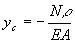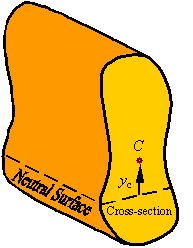Bending moment and its relation to radius of curvature:The bending moment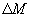about the neutral surface that is created by the normal loadresulting from the normal stress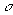acting on the areaof the cross section can be calculated byIntegrating over the cross section to get the total moment transmitted through the cross section gives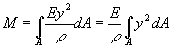Recalling that the integral in this relation is the area moment of inertial I about the neutral axis (the line resulting from the intersection of the cross section and the neutral surface), the relation between the bending moment M and radius of curvature r of the neutral axis of the beam becomes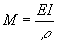From this relation one can calculate the expression for stress as a function of the bending moment by substituting in the expression for axial stress this relation for the radius of curvature. This gives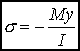As can be seen in the figure, the maximum and minimum normal stresses occur in the material that is furthest away from the neutral surface (either at the top or bottom of the bar depending on the actual direction of the moment).